Courses

# RD Sharma Solutions Ex-1.3, Number System, Class 9, Maths Class 9 Notes | EduRev

## RD Sharma Solutions for Class 9 Mathematics

Created by: Abhishek Kapoor

## Class 9 : RD Sharma Solutions Ex-1.3, Number System, Class 9, Maths Class 9 Notes | EduRev

The document RD Sharma Solutions Ex-1.3, Number System, Class 9, Maths Class 9 Notes | EduRev is a part of the Class 9 Course RD Sharma Solutions for Class 9 Mathematics.
All you need of Class 9 at this link: Class 9

Q1. Express each of the following decimals in the form of rational number.

(i) 0.39

(ii) 0.750

(iii) 2.15

(iv) 7.010

(v)  9.90

(vi) 1.0001

Solution:

(i) Given,

0.39 = 39/100

(ii) Given,

0.750 = 750/1000

(iii) Given,

2.15 = 215/100

(iv) Given,9.101

(iv)  Given,

7.010 = 7010/1000

(v) Given,

9.90 = 990/100

(vi) Given,

1.0001 = 10001/10000

Q2. Express each of the following decimals in the form of rational number (p/q)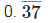(i)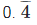(ii)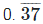Solution:

(i) Let x =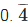Then, x =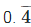= 0.444…      ___ (a)

Multiplying both sides of equation (a) by 10, we get,

10x = 4.44....           ___ (b)

Subtracting equation (1) by (2)

9x = 4

x = 4/9

Hence,= x = 4/9

(ii) Let x =Then, x == 0.3737…      ___ (a)

Multiplying both sides of equation (a) by 100, we get,

100 x = 37.37....                                                              ___ (b)

Subtracting equation (1) by (2)

99 x = 37

x = 37/99

Hence,= x = 37/99

91 docs

,

,

,

,

,

,

,

,

,

,

,

,

,

,

,

,

,

,

,

,

,

,

,

,

,

,

,

,

,

,

;